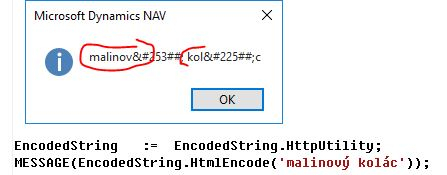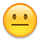Howdy, Stranger!

It looks like you're new here. If you want to get involved, click one of these buttons!

I have a string like "sample". I need to get a string of it in hex format With DotNET from C/AL

edited 2018-11-05
Good morning everyone,

I have a string like "sample". I need to get a string of it in hex format; like this:

%48%65%6C%6C%6F%20%57%6F%72%6C%64%21 <=> 'Hello World!'

I need a metod that let me Conversion from and to hexadecimal format. I'm programming on the Dynamics Nav 2015 platform.

Thank you so much in advance

Michele

• Why C/AL is not enough and you need .NET based solution?
``````FOR i := 1 TO STRLEN(InText) DO BEGIN
//c, UpperByte, LowerByte : Integer
//UpperByteChar, LowerByteChar : Char
c := InText[i];
UpperByte := c DIV 16;
LowerByte := c - (UpperByte * 16);

IF LowerByte < 10 THEN
LowerByteChar  := 48 +LowerByte
ELSE
LowerByteChar  := 87 +LowerByte;

IF UpperByte < 10 THEN
UpperByteChar := 48+UpperByte
ELSE
UpperByteChar := 87+UpperByte;

HexText += STRSUBSTNO('%%1%2', UpperByteChar, LowerByteChar);
END;
``````

Because I prefer to exploit the potential that the dotNet platform offers, simply this. Thank you so much,

Michele

``````string input = "Hello World!";
char[] values = input.ToCharArray();
foreach (char letter in values)
{
// Get the integral value of the character.
int value = Convert.ToInt32(letter);
// Convert the decimal value to a hexadecimal value in string form.
string hexOutput = String.Format("{0:X}", value);
Console.WriteLine("Hexadecimal value of {0} is {1}", letter, hexOutput);
}
/* Output:
Hexadecimal value of H is 48
Hexadecimal value of e is 65
Hexadecimal value of l is 6C
Hexadecimal value of l is 6C
Hexadecimal value of o is 6F
Hexadecimal value of W is 57
Hexadecimal value of o is 6F
Hexadecimal value of r is 72
Hexadecimal value of l is 6C
Hexadecimal value of d is 64
Hexadecimal value of ! is 21
*/
``````

• edited 2018-11-02
"You can’t just ask customers what they want and then try to give that to them.
By the time you get it built, they’ll want something new.” Steve Jobs
•HtmlEncode method of the HttpUtility calss doesn't allow to convert the whole string in hexadecimal format but converts only a part of itThank you so much in advance

Michele

• Are there any other updates?

Thank you so much in advance

Michele
• edited 2018-11-05
Why C/AL is not enough and you need .NET based solution?
``````FOR i := 1 TO STRLEN(InText) DO BEGIN
//c, UpperByte, LowerByte : Integer
//UpperByteChar, LowerByteChar : Char
c := InText[i];
UpperByte := c DIV 16;
LowerByte := c - (UpperByte * 16);

IF LowerByte < 10 THEN
LowerByteChar  := 48 +LowerByte
ELSE
LowerByteChar  := 87 +LowerByte;

IF UpperByte < 10 THEN
UpperByteChar := 48+UpperByte
ELSE
UpperByteChar := 87+UpperByte;

HexText += STRSUBSTNO('%%1%2', UpperByteChar, LowerByteChar);
END;
``````
Slawek Guzek
Dynamics NAV, MS SQL Server, Wherescape RED;
GDPR Certified Data Protection Officer - PECB License DPCDPO1025070-2018-03
• Why C/AL is not enough and you need .NET based solution?
``````FOR i := 1 TO STRLEN(InText) DO BEGIN
//c, UpperByte, LowerByte : Integer
//UpperByteChar, LowerByteChar : Char
c := InText[i];
UpperByte := c DIV 16;
LowerByte := c - (UpperByte * 16);

IF LowerByte < 10 THEN
LowerByteChar  := 48 +LowerByte
ELSE
LowerByteChar  := 87 +LowerByte;

IF UpperByte < 10 THEN
UpperByteChar := 48+UpperByte
ELSE
UpperByteChar := 87+UpperByte;

HexText += STRSUBSTNO('%%1%2', UpperByteChar, LowerByteChar);
END;
``````

Because I prefer to exploit the potential that the dotNet platform offers, simply this. Thank you so much,

Michele
Why C/AL is not enough and you need .NET based solution?
``````FOR i := 1 TO STRLEN(InText) DO BEGIN
//c, UpperByte, LowerByte : Integer
//UpperByteChar, LowerByteChar : Char
c := InText[i];
UpperByte := c DIV 16;
LowerByte := c - (UpperByte * 16);

IF LowerByte < 10 THEN
LowerByteChar  := 48 +LowerByte
ELSE
LowerByteChar  := 87 +LowerByte;

IF UpperByte < 10 THEN
UpperByteChar := 48+UpperByte
ELSE
UpperByteChar := 87+UpperByte;

HexText += STRSUBSTNO('%%1%2', UpperByteChar, LowerByteChar);
END;
``````

Because I prefer to exploit the potential that the dotNet platform offers, simply this. Thank you so much,

Michele

``````string input = "Hello World!";
char[] values = input.ToCharArray();
foreach (char letter in values)
{
// Get the integral value of the character.
int value = Convert.ToInt32(letter);
// Convert the decimal value to a hexadecimal value in string form.
string hexOutput = String.Format("{0:X}", value);
Console.WriteLine("Hexadecimal value of {0} is {1}", letter, hexOutput);
}
/* Output:
Hexadecimal value of H is 48
Hexadecimal value of e is 65
Hexadecimal value of l is 6C
Hexadecimal value of l is 6C
Hexadecimal value of o is 6F